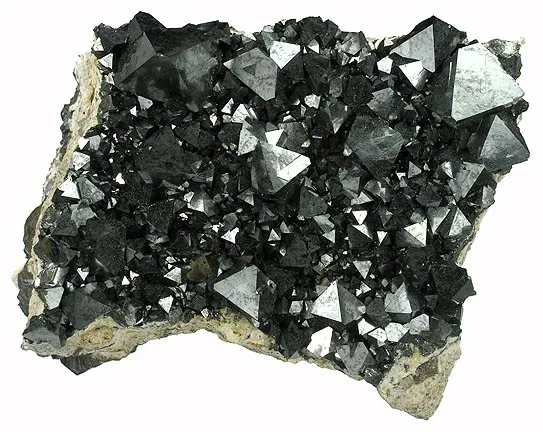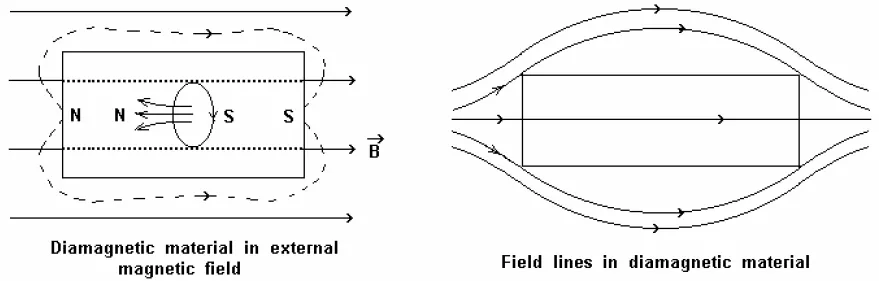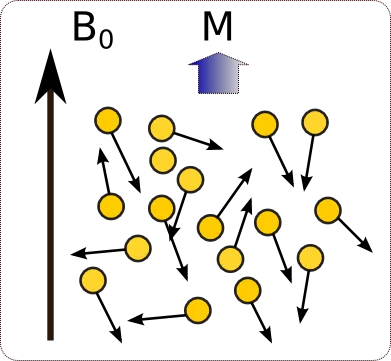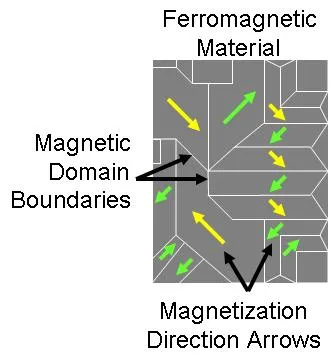# Physics - Electromagnetism - Magnetic MaterialsImage source:## Introduction

Hello it's a me again Drifter Programming! Today we continue with Electromagnetism to get into Magnetic Materials. So, without further do, let's get straight into it!

## Magnetic materials

Magnetic materials are materials studied and used mainly for their magnetic properties. The magnetic response of a materials is largely determined by the magnetic dipole moment associated with the intrinsic angular momentum, or spin, of its electrons. A material’s response to an applied magnetic field can be characterized as diamagnetic, paramagnetic, ferromagnetic or antiferromagnetic.

From nature.com

## Classification/Types of Magnets

### Diamagnetism

The fundamental property of all matter is Diamagnetism. It's caused by the non-cooperative behavioral orbiting of electrons when exposed to an magnetic field. Such substances are composed of atoms that have no net magnetic moments, but get magnetized when being affected by an magnetic field (negative magnetization). From all that we can clearly see that the total magnetization is zero, when the magnetic field is zero. A characteristic behavior of diamagnetic materials is the so called  susceptibility which is temperature independent. Susceptibility means that an extensive property is not affected by the variation of an intensive property (temperature in our case). This means that the effect of an magnetic field to an diamagnetic is not affected by the temperature. Calculating the "interaction" for any temperature gives us "one and only" result.Image source:Diamagnetic materials repel any externally applied magnetic field. A usually weak force for most materials that opposes the magnetic field by realigning . Electrons within atoms of diamagnetic materials are paired (individual atomic dipoles) and therefore don't generate their own magnetic field, which explains why the total net magnetic moment is zero. Permanent magnetic dipoles orient them self-parallel to the applied magnetic field and give rise to a positive magnetization, since the orientation of the dipoles parallel to the applied magnetic field is not complete, the magnetization is therefore very small. Also note that most elements of the periodic table are diamagnetic.

### Paramagnetism

Paramagnetic materials on the other hand have unpaired electrons and so a net magnetic moment/field which is not zero. Those individual moments of each unpaired electron do not interact and so the total magnetization is still zero when the paramagnetic material is not affected by a magnetic field (similar to Diamagnetic materials). The thing that clearly changes is the orientation/alignment to the magnetic field. We now have a partial alignment, cause only the paired electrons are affected and so now have a positive magnetization. This alignment depends of the temperature changes which means that we have a temperature dependent susceptibility (known as Curie's law). For "normal" temperature this paramagnetic susceptibility is small, but still larger then the diamagnetic one. For very low temperatures, a high paramagnetic susceptibility is independent of the applied magnetic field.Image source:From all that we covered till now we can see that paramagnetic materials are far less affected by magnetic fields, but they still maintain their magnetic properties when no magnetic field is applied to them. Many elements are paramagnetic, but because the attractive force is much smaller they sometimes are considered "non-magnetic". Such materials can only be magnetized by strong magnetic fields and act in the direction where this magnetic field is applied (opposite direction). Paramagnetic materials thereforce move from weaker to stronger parts of the applied magnetic field.

### Ferromagnetism

In paramagnetic materials the magnetic moments do not interact. In ferrymagnetic materials these moments exhibit very strong interactions. The interactions between those moments are produced by electronic exchange forces and result in a parallel or antiparallel alignment. Those forces are very large and equivalent to a field of 1000Tesla! This is quantum mechanical phenomenon due to the relative orientation of the spins of two electron. From all that we can clearly see that we have a very large net magnetization (not zero like in the other two types we've seen before) even when no external magnetic field is present. Two distinct characteristics of ferromagnetic materials are their spontaneous magnetization and the existence of magnetic ordering temperature. Spontaneous magnetization is caused by the net magnetization that exists inside even at the absence of a field. A related term is the so called saturation magnetization, which we can measure in a lab and is dependent of the temperature, buy independent of the particle size. After a maximum induced magnetic moment is obtained, the field can no further increase when magnetization occurs. Ferromagnets can retain a memory of an applied field once it is removed. This behavior is called hysteresis and a plot of the variation of magnetization with magnetic field is called a hysteresis loop.Image source:Ferromagnetic materials are classified into two types:

1. Soft Ferromagnetic materials -> High relative permeability, low coercive force, easily magnetized and demagnetized and have extremely small hysteresis.
2. Hard Ferromagnetic materials -> Low permeability, very high coercive force, difficult to magnetize and demagnetize. They also retain high percentage of their magnetization and have relatively high hysteresis loss.

The so called Antiferromagnet shows a behavior of susceptibility above a critical temperature which is the so called Neel temperature (Tn). Above this temperature such magnetic materials obey the Curie-Weiss law of paramagnets, but with an negative interception caused by negative exchange interactions.

### Ferrites

A special group of ferromagnetic materials that have ferromagnetic and antiferromagnetic properties are the Ferrites. They have a high permeability and are held by binding resin. The magnetization caused by the unpaired electrons is large enough to be conserned, but the magnetic saturation is not as high as in ferromagnetic materials. Ferrites are split into soft and hard ones, similar to ferromagnets as following:

1. Soft Ferrites -> Square hysteresis loop, high resistance and demagnetization. The most important property is their high resistivity.
2. Hard Ferrites ->  These materials have a hexagonal structure and low in cost and density.

### Ferrimagnetism

Ionic combounds (such as oxids) have a crystal structure which causes complex forms of magnetic ordering. One type of magnetic ordering is called ferrimagnetism. The magnetic spins of a ferrimagnetic oxide is as shown:Image source:The magnetic structure is composed by two magnetic sublattices called A and B separated by oxygens and the exchange are mediated by the oxygen anions. These exchanges are called indirect or superexchange interactions. The strongest superexchange interactions  result in an antiparallel alignment os spins between the A and B sublattice. The magnetic moments of A and B are unequal and result in a net magnetic moment. Similar to ferromagnetism we again have spontaneous magnetization, Curie temperatures, hysteresis and remanence. The only thing that changes is the very different magnetic ordering of the atoms..

## Magnetic material examples

Let's get into examples of magnetic materials in nature for each of the previous types/classes that we explained in "theory".

Familiar diamagnets are:

• bismuth
• phosphorus
• antimony
• copper
• water
• alcohol
• hydrogen

Familiar paramagnets are:

• aluminum
• maganese
• platinum,
• crown glass
• solution of salts of iron and oxygen

Familiar ferromagnets are:

• iron
• nickel
• cobalt and their alloys

Ferrites are:

• pure iron
• Compounds consisting of strontium carbonate or barium and iron oxide (Fe2O3)

A simple example of an ferrimagnet is Magnetite. Fe304 crystalized in a spinel structure and has large oxygen ions that are closely packed in cubis arrangements of smaller Fe ions that fill the gabs.Image source:## REFERENCES:

### Electric fields:

Getting into Electromagnetism -> electromagnetim, electric charge, conductors, insulators, quantization

Coulomb's law with examples -> Coulomb's law, superposition principle, Coulomb constant, how to solve problems, examples

Electric fields and field lines -> Electric fields, Solving problems around Electric fields and field lines

Electric dipoles -> Electric dipole, torque, potential and field

Electric charge and field Exercises -> examples in electric charges and fields

### Electric flux:

Electric flux and Gauss's law -> Electric flux, Gauss's law

Applications of Gauss's law (part 1) -> applying Gauss's law, Gauss applications

Applications of Gauss's law (part 2) -> more Gauss applications

Electric flux exercises -> examples in electric flux and Gauss's law

### Electric potential:

Electric potential energy -> explanation of work-energy, electric potential energy

Calculating electric potentials -> more stuff about potential energy, potential, calculating potentials

Millikan's Oil Drop Experiment -> Millikan's experiment, electronvolt

Cathode ray tubes explained using electric potential -> cathode ray tube explanation

Electric potential exercises (part 1) -> applications of potential

Electric potential exercises (part 2) -> applications of potential gradient, advanced examples

### Capacitance:

Capacitors (Condensers) and Capacitance -> Capacitors, capacitance, calculating capacitance

How to solve problems around Capacitors -> combination, solving problems, simple example

Electric field energy and density -> Electric field energy, energy density

Dielectric materials -> Dielectrics, dielectric constant, permittivity and strength, how to solve problems

Electric capacitance exercises -> examples in capacitance, energy density and dielectrics

### Current, resistance and EMF:

Electric current -> Electric current, current density

Electrical resistivity and conductivity -> Electrical resistivity, conductivity, thermal coefficient of resistivity, hyperconductivity

Electric resistance -> Resistance, temperature, resistors

Electromotive Force (EMF) and Internal resistance -> Electromotive force, internal resistance

Power and Wattage of Electronic Circuits -> Power in general, power/wattage of electronic circuits

Electric current, resistance and emf exercises -> exampes in all those topics

### Direct current (DC) circuits:

Resistor Combinations -> Resistor combinations, how to solve problems

Kirchhoff's laws with applications -> Kirchhoff's laws, how to solve problems, applications

Electrical measuring instruments -> what are they?, types list, getting into some of them, an application

Electronic circuits with resistors and capacitors (R-C) -> R-C Circuit, charging, time constant, discharging, how to apply

RC circuit exercises -> examples in Kirchhoff, charging, discharging capacitor with/without internal resistance

### Magnetic field and forces:

Magnetic fields -> Magnetism, Magnetic field

Magnetic field lines and Gauss's law of Magnetism -> magnetic field lines, mono- and dipoles, Flux, Gauss's law of magnetism

The motion of charged particles inside of a magnetic field -> straight-line, spiral and helical particle motion

Applications of charged particle motion -> CERN, Cyclotrons, Synchrotrons, Cavity Magetron, Mass Spectrometry and Magnetic lens

Magnetic force applied on Current-Carrying Conductors -> magnetic force on current-carrying conductors/wires, proofs

Magnetic force and torque applied on current loops (circuits) -> magnetic force on current loops, magnetic moment and torque

Explaining the Physics behind Electromotors -> tesla, history and explaining the physics behind them

Magnetic field exercises -> examples in magnetic force, magnetic flux, particle motion and forces/torque on current-carrying conductors

### Magnetic field sources:

Magnetic field of a moving charged particle -> moving charge, magnetic field, force between parallel charged particles

Magnetic field of current-carrying conductors -> magnetic field of current, Biot-Savart law

Force between parallel conductors and the magnetic field of a current loop -> force between parallel conductors, magnetic field of current loop

Ampere's law and Applications -> Ampere's law, applications

And this is actually it for today's post! Next time we will get into Displacement currnet, which is closely binded to Ampere's law!

Bye!

H2
H3
H4
3 columns
2 columns
1 column